Applied and Computational Mathematics
Volume 5, Issue 2, April 2016, Pages: 78-82

Tuncay Can’s Approximation Method to Obtain Initial Basic Feasible Solution to Transport Problem

Tuncay Can, Habip Koçak

Department of Econometrics, Faculty of Economics, Marmara University, Istanbul, Turkey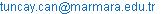(T. Can)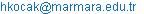(H. Koçak)

Tuncay Can, Habip Koçak. Tuncay Can’s Approximation Method to Obtain Initial Basic Feasible Solution to Transport Problem. Applied and Computational Mathematics. Vol. 5, No. 2, 2016, pp. 78-82. doi: 10.11648/j.acm.20160502.17

Received: April 14, 2016; Accepted: April 27, 2016; Published: May 12, 2016

Abstract: Obtaining an initial basic feasible solution to a transport problem – or a corner point in the convex polytope region – is extremely important in terms of reaching the optimal solution to the problem in the shortest time. When a transport problem is basically accepted as a linear programming problem, a degenerated solution is caused by the structure of the simplex method used when modelling with linear programming and located in a corner point sometimes at the optimal solution itself but mostly in close proximity to the optimal solution vector. One of the ways to eliminate this degenerated solution is to employ approximation methods. The main aim of this paper is to introduce Tuncay Can’s approximation method, which was developed as an alternative to the approximation methods in the literature for a balanced transport problem. Tuncay Can’s approximation method usually has less iterations than other approximation methods. In this paper, the Tuncay Can approximation method is introduced as an alternative to The North West Corner Rule, Minimum Cost Method, and the RAM and VAM methods.

Keywords: Basic Feasible Solution, Transportation Problems, Can Method, VAM, RAM

Contents

1. Introduction

The most generic statement of a transport problem as a linear programming problem can be stated mathematically as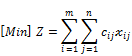subject to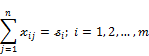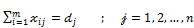(1)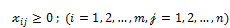In this model, m is the number of production centres and n is the number of consumption centres;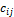gives the cost of a product that will be transported fromproduction centre toconsumption centre, while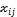gives the number of products to be transported fromto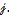When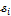represents the supply from the production centreand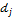represents the demand from to the consumption centre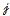, then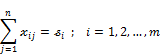shows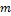number of supply constraints and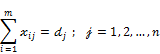shows n number of demand restraints. Therefore, there are a total of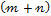supply and demand restraint conditions in thetransport problem. If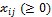,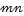number of unknowns or decision variables are defined, called ‘obligatory restraints’. In this case the number of equations isand the number of variable is.

A transport problem is generally represented with a transport tableau.

Table 1. Transport Tablesu.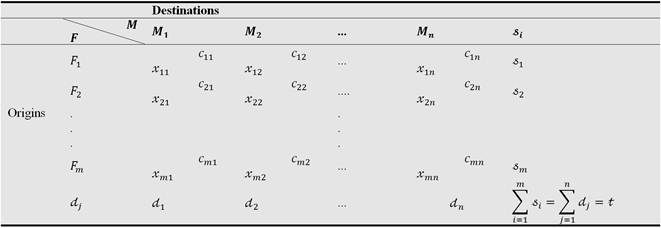A transport problem where total supply is equal to total demand is called a balanced transport problem; otherwise, it is an unbalanced transport problem. Definitions, theorems for transport models, in other words, the internal dynamics of a transport problem, are built on balanced transport problems; thus, the Tuncay Can approximation method, which will be explained and introduced in this paper, will be shown for a balanced transport problem. The principle assumed is that every unbalanced transport problem can always be converted to a balanced transport problem by adding an artificial production centre or consumption centre.

In the system defined with (1), each of thedecision variables in the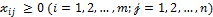inequality represents closed half-space in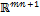when the transport model is handled in its entirety and the region shown by the closed half-space mentioned is a non-negative region. This generally shows the region where the convex polytope region consists, which is the geometric picture of a transport problem in general. Theconstraints handle the region to be studied in the transport problem. For this reason, leaving these constraints aside, there is a constraint in the form of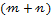number of equations in system (1), where each constraint is hyperplane.

In order to solve system (1) with the simplex method, that is, to start iteration – in other words to obtain a basic feasible solution (a corner point) – the rank of the matrix consisting of the coefficients ofunknowns created by the supply and demand constraint in system (1) has to be. This rank beingguarantees the existence oflinear independent equations, which means thatbasic variables are non-zero and positive and all other non-basic variables are zero. As a result of this, instead of a non-basic feasible solution vector with infinite numbers (solution without corner point) in the convex polytope region that emerges in the relevant dimension, a basic feasible solution with finite numbers (corner points) is obtained. However, in a balanced transport problem, as a result of the equation of total supply to total demand, a lack of necessity for any equation in system (1) emerges, and it becomes clear that the rank of the coefficients matrix cannot be. This means thatlinear independent equations cannot exist in the relevant equation system, which knocks out the starting condition of iteration in a solution with the simplex method. In other words, thebasic variables must be non-zero and positive, and the other, non-basic variables must be zero, which is a condition for starting corner point location for a journey between convex points in a corner polytope region – and this leads to more basic variables than whenis zero, which is degeneration.

Definition: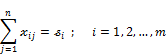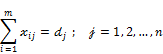The rank of the above system is exactly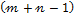. The number of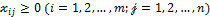basic variables is exactly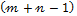. 

In that case, since a degenerated solution consists, instead of the simplex method, which generally solves linear programming problem in the most effective manner, it is guaranteed as expressed in the definition thatbasic variables be non-zero and positive and other non-basic variables be zero, taking into consideration supplies and demands in a balanced transport problem; thus, the initial basic feasible solution or initial distribution plan is obtained with approximation methods.

The geometric picture of the initial basic feasible solutionreveals a corner point that is not optimal but relatively remote or close to the optimal solution according to approximation methods generally in the emerging convex polytope region when obligatory constraints are neglected, constraint conditions which are hyperplanes and, again, the hyperplane objective function is taken into consideration – which means that transport problem is considered in its entirety.

In order to obtain the basic feasible solution, the most widely known methods in the literature may be listed as North West Corner Rule, Minimum Cost Method, and the RAM and VAM methods.

2. Literature Search

Recently, a consideration of the effectiveness of approximation methods in the literature and efforts to develop new methods have been witnessed. Clearly, Vogel’s Approximation Method (VAM) is effective in terms of proximity to optimal result. For this reason, newly developed techniques have been introduced, especially  related to VAM. Kırca and Satır  compared the total opportunity costs intuitive method that they developed and VAM for 480 examples in their 1990 dated paper ‘A Heuristic for Obtaining an Initial Solution for the Transport Problem’, and in 134 examples the developed method reached optimal result directly while VAM could not, and it used computer time 34-45% more effectively. In 2009, Krishnaswamy et al. developed an intuitive method which was based on this , and this improved VAM (IVAM) method produced even more optimal results.

Korukoğlu and Ballı, compared Krishnaswamy et al.’s IVAM and VAM for 1000 examples that they developed and also found that this IVAM was more effective than VAM in terms of performance and usage of computer time . Sood and Jaid compared VAM with the Maximum Differences method that they developed and found out in their study that in some examples their proposed method had advantages over VAM and that it produced results that were more feasible to the optimal .

However, the most criticized aspect of such comparative advantage-finding problems is the low number of examples. In particular, simple examples and those with single-tests are insufficient to determine the advantages of one technique over another. Singh et al. compared VAM with IVAM variants in their 2012 research, performing example tests at 12 different sizes to determine the IVAM advantages .

In his 1990 dated study, Balakrishnan developed the VAM method for unbalanced transport problems and concluded that it is a more effective method . Juman and Hoque developed a more effective initial solution method with the help of a Minimum Total Cost Solution method that they developed in 2015 .

In this paper, the Tuncay Can Approximation Model will be introduced as an alternative to the above mentioned approximation methods.

3. Tuncay Can’s Approximation Method

Below is the algorithm of the approximation method developed in early 2015 by Tuncay Can with the purpose of obtaining an initial basic feasible solution vector (a corner point) so as to reach an optimal solution in a balanced transport problem :

Step 1: In a balanced transport problem, assuming that there are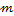production centres and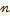consumption centres, the unit transport costs of one unit good to be transported from each centre of production to each centre of consumption(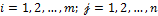), the geometric mean of unit transport costs isThe transport cost that is the closest unit to the value found with this geometric mean in terms of absolute value is determined. If the closest unit transport costs are equal, then one of them is chosen randomly. The cell including the mentioned unit transport cost is filled with the maximum load, taking into account the supply (rows) and demand (columns). If the supply is exactly met, the relevant row is deleted from the transport tableau; if the demand is met, the relevant column is deleted from the transport tableau. In certain conditions, however, both of these are not deleted at the same time.

Step 2: As each term of the objective function is one of the dynamics of the transport problem – in other words, as the decision variables that employ the terms and relevant unit transport costs are independent from one another – the geometric mean of unit transport costs applied in Step 1 is taken and relevant cell is loaded. As a result of this, either the supply (row) or demand (column) will be met totally and the relevant row or column is deleted from the tableau; then, the geometric mean of the remaining unit transport costs is taken again and the procedure given in Step 1 is applied.

Step 3: If there are only two unit transport costs remaining, there is no problem with taking geometric mean for the mentioned costs; however, the maximum possible distribution is made taking into consideration the supply (row) and demand (column) for that with the smallest unit transport cost.

Step 4: Steps 1, 2 and 3 are applied until an initial basic feasible solution to the balanced transport problem is obtained.

In Tuncay Can’s approximation method, which is based on Cauchy’s inequality, the preference for the geometric mean is due to the fact that relative deviations around the central tendency in the geometric mean are symmetric and that it is less affected by extreme values than is the arithmetic mean. Again, in the introduced approximation method, unit transport costs being the coefficients of decision variables in the terms of the objective function of a transport problem will ensure that the geometric mean is located in a closer area to the optimal corner point of the hyperplane created with the value attached to the objective function relevant to the geometric mean of coefficients.

The algorithm provided for Tuncay Can’s approximation method can also be applied to unbalanced transport problems. As noted (above), an unbalanced transport problem can be converted to the form of a balanced transport problem by adding an artificial production or consumption centre, depending on the deficiency. As the unit transport cost of artificial centres is zero, when Tuncay Can’s approach is applied to unbalanced transport problems, the fact that the initial geometric mean is zero and that making the maximum distribution possible taking into account the supply (row) and demand (column) to this cell does not pose a problem for the algorithm can be seen as an advantage, since it operates against the possibility that the cost in a relevant cell can be made zero and cause a reduction in total cost.

In order to ensure that the algorithm introduced for Tuncay Can’s approximation method is valid, which means that an initial solution vector thus obtained be secured to correspond to a corner point in the convex polytope region (which is the geometric picture of transport problem), it needs to be shown that (i) the point obtained with Tuncay Can’s approximation method is the feasible solution vector, and that (ii) this indicates a corner point. In order to obtain such proof, the general structure of the transport problem is considered again.

Let us take into consideration the structure of the linear programming problem of a balanced transport problem, as defined with (1). When the coefficients of decision variables in supply and demand constraints are organized in harmony with their indices, it can be seen that they consist of only the numbers ‘0’ and ‘1’. With matrices that correspond to the coefficients matrix generated by the integers ‘0’ and ‘1’, every transport problem can be organised in upper triangular, lower triangular or triangular forms. In addition, as the rank of the mentioned matrix is, it can be proved with the Basic Triangularity Theorem that every basic solution of the transport problem is triangular:

3.1. Theorem 1 (Basic Triangularity Theorem)

Every basic solution of transport problem is triangular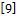.

The Basic Triangularity Theorem is not a structure shaped according to the algorithm of approximation methods, but it emphasises the structure at the core of the transport problem. Generally, ‘basic solutions’ is a general term covering all the corner points inside and outside the convex polytope region. For this reason, a feasible solution that includes the corner points in the convex polytope region should be ensured. A feasible solution and Tuncay Can’s approximation method can be combined to give the following theorem and its proof.

3.2. Theorem 2

The solution obtained with Tuncay Can’s approach always has a feasible solution.

Proof : Generally, a balanced transport problem has always a feasible solution. For this effect, because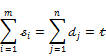then for each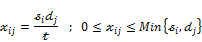variables generate a feasible solution.

In reality, when the equation system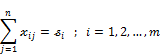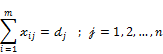is taken into consideration, it must be shown that the following variables emphasised as a feasible solution

provide the equation system for eachand. Under the assumption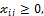the following equation is verified. Then,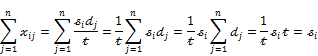so the accuracy of the following relation is shown: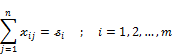Again, taking into account the assumptionthe following equation is also verified: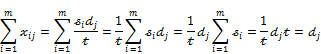Therefore, as this relation is also verified,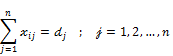it follows that the balanced transport problem always has a feasible solution.

Then, as every balanced transport problem has a feasible solution regardless of the approximation method employed, the solution obtained with Tuncay Can’s approximation method is naturally a feasible one and located in the convex polytope region in the space with the relevant dimension.

If there is a feasible solution, there also is an optimal solution . It is important that the obtained solution vector is located in the feasible solution zone. However, what is much more important is to prove that the relevant solution vector is the feasible solution, meaning that it is a corner point in the convex polytope region with relevant dimension. Before giving the last theorem and its proof related to the foregoing, the triangularity algorithm rule  must be mentioned.

The triangularity rule is an algorithm that was developed in order to obtain an initial basic feasible solution to a transport problem in the simplest manner. In this algorithm, a variable is selected as the candidate for the first feasible basic variable and, taking into consideration the relevant supply and demand (column and row), the maximum possible distribution is made to the cell with the chosen basic variable. If the supply is totally met, the relevant row is deleted from the tableau, and if the demand is totally met, the relevant column is deleted. The steps of the algorithm continue until a basic feasible solution is found. The triangularity rule is a general one. All approximation methods are special forms of the triangularity rule, with their unique algorithms. Naturally Tuncay Can’s approximation method is based on the geometric mean in its algorithm, and it is also a special form of the triangularity rule.

3.3. Theorem 3

The solution obtained with Tuncay Can’s approximation method is an initial basic feasible solution, which means that it is a corner point of the convex polytope.

Proof: From Theorem 2, the solution obtained with Tuncay Can’s approximation method always has a feasible solution. As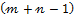basic variables in the system denoted with (1) in a balanced transport problem are non-zero and positive, in every balanced transport problem a basic feasible solution, meaning a corner point, is obtained. Every solution determined with the triangularity rule is a basic feasible solution  and Tuncay Can’s approximation method is also a basic feasible solution, meaning that it is a corner point of the convex polytope region.

4. Conclusion

Obtaining an initial basic feasible solution for a balanced transport problem is extremely important as the simplex method provides degenerated solutions and the algorithms written to avoid this degenerated solution are so long that emerges as a problem in reaching the optimal solution. Approximation methods test whether or not the convex polytope in a transport problem has a corner point. If it is optimal then, there is no problem, but if the relevant corner point is not optimal, then the journey between corner points includes an optimality test at each instance and continues until an optimal point is found.

The main aim of this paper is to introduce Tuncay Can’s approximation method, which was developed as an alternative to the approximation methods in the literature for a balanced transport problem. Tuncay Can’s approximation method usually has less iterations than other approximation methods, which derives from its following algorithm steps and placement in the corner point in the convex polytope region, which is the geometric reflection of a transport problem in the form of linear programming, until the optimal point is reached. A comparison between approximation methods is outside the scope of this paper; this comprises the subject of another article.

References

1. T. Can, "Yöneylem Araştırması, Nedensellik Üzerine Diyaloglar I", Beta Publications, Istanbul, p. 396, 2015.
2. M., Kırca, A. Satır, "A Heuristic for Obtaining and Initial Solution for the Transportation Problem", Journal of the Operational Research Society, Vol. 41, No. 9, pp. 865-871, 1990.
3. K. N., Krishnaswamy, A. I., Sivakumar, M., Mathirajan, Management Research Methodology, Dorling Kindersley Pvt. Ltd., p. 246, 2009.
4. S. Korukoğlu, S., Ballı, "An Improved Vogel’s Approximation Method for the Transportation Problem", Mathematical and Computational Applications, Vol. 16, No. 2, pp. 370-381, 2011.
5. S., Sood, K., Jain, "The Maximum Difference Method to find Initial Basic Feasible Solution For Transportation Problem", Asian Journal of Management Sciences, 03 (07), pp. 8-11, 2015.
6. S., Singh, G. C., Dubey, R., Shrivastava, R, "Optimization and Analysis of Some Variants Through Vogel’s Approximation Method (VAM)", IOSR Journal of Engineering, Vol. 2, Issue 9, pp. 20-30, 2012.
7. N., Balakrishnan, "Modified Vogel’s Approximation Method for the Unbalanced Transportation Problem", Applied Mathematics Letters, Vol. 3, Issue. 2, pp. 9-11, 1990.
8. Z. A. N. S., Juman, M. A., Hoque, "An Efficient Heuristic to Obtain a Better Initial Feasible Solution to the Transportation Problem", Applied Soft Computing , Vol. 34 , pp. 813-826, 2015.
9. B. G., Dantzig, N. M., Thapa, Linear Programming 1. Introduction, Springer-Verlag New York, p. 214, 1997.
10. M. Zangiabadi, T. Rabie," A New Model for Transportation Problem with Qualitative Data", Iran. Journal of Operations Research, 3 (2), pp. 33–46 (2012).
11. V. J. Sudhakar, N. Arunsankar, T. Karpagam, "A New Approach for Finding An Optimal Solution for Transportation Problems, Europan Journal of Sciences Research, 68 (2), pp. 254–257, 2012.
12. V. Srinivasan, G. L. Thompson, "Cost Operator Algorithms for the Transportation Problem", Mathematical Programming, 12, pp. 372–391, 1977.
13. N. Sen, T. Som, B. Sinha, "A Study of Transportation Problem for an Essential Item of Southern Part of North Eastern Region of India as an OR Model and Use of Object Oriented Programming", International Jorunal of Computation Sciences Network Security, 10 (4), pp. 78-86, 2010.
14. F. Pargar, N. Javadian, A. P. Ganji, "A Heuristic for Obtaining an Initial Solution for the Transportation Problem with Experimental Analysis", in: The 6th International Industrial Engineering Conference, Sharif University of Technology, Tehran, Iran, 2009.
15. M. Mathirajan, B. Meenakshi, "Experimental Analysis of Some Variants of Vogel’s Approximation Method", Asia-Pacian. Journal of Operations Research, 21 (4), p. 447–462, 2004.

 Contents 1. 2. 3. 3.1. 3.2. 3.3. 4.
Article ToolsAbstractPDF(203K)